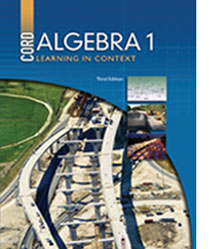# Algebra 1 - 3rd Edition

## Chapter 5: Nonlinear Functions

Some links are repeated for use with more than one lesson.

### 5.1 Relations and Functions

http://www.mathwarehouse.com/algebra/relation/math-function.php
This site explains relations and functions and has practice problems.

http://www.purplemath.com/modules/fcns.htm
This page contains a written lesson explaining the differences between relations and functions and how to tell them apart.

### 5.2 Evaluating Functions

http://www.algebralab.org/lessons/lesson.aspx?file=Algebra_FunctionsRelationsEvaluation.xml
This page contains a description of how to evaluate functions with practice problems.

http://www.sparknotes.com/testprep/books/newsat/chapter19section11.rhtml
This site explains how to evaluate functions and how to use them for different operations.

### 5.3 Direct and Inverse Variation

http://www.explorelearning.com/index.cfm?method=cResource.dspDetail&ResourceID=129
This page contains a gizmo that has a graph displaying direct and inverse variation.

http://hotmath.com/help/gt/genericalg1/section_9_8.html
This site contains practice problems for direct and inverse variation.

### 5.5 Functions Involving Square Roots

http://www.explorelearning.com/index.cfm?method=cResource.dspDetail&ResourceID=107
This site contains a gizmo that explores functions involving square roots.

### 5.6 Exponential Growth and Decay

http://www.regentsprep.org/regents/math/algebra/AE7/ExpDecayL.htm
This page explains exponential growth and decay with notes, tables, and graphs.

http://hotmath.com/help/gt/genericalg1/section_9_6.html